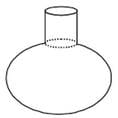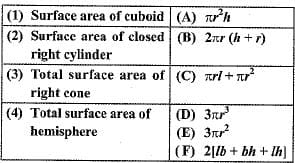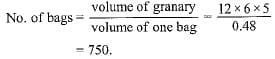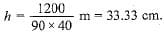# Important Questions: Surface Area & Volumes

## 10 Questions MCQ Test NCERT Mathematics for CAT Preparation | Important Questions: Surface Area & Volumes

Description
Attempt Important Questions: Surface Area & Volumes | 10 questions in 10 minutes | Mock test for Class 10 preparation | Free important questions MCQ to study NCERT Mathematics for CAT Preparation for Class 10 Exam | Download free PDF with solutions
QUESTION: 1

Solution:
QUESTION: 2

### A surahi is the combination of

Solution:QUESTION: 3

### A cubical ice cream brick of edge 22 cm is to be distributed among some children by filling ice cream cones of radius 2 cm and height 7 cm upto its brim. How many children will get the ice cream cones?

Solution:
QUESTION: 4

Match the columnSolution:
QUESTION: 5

The volume of the largest right circular cone that can be cut out from a cube of edge 4.2 cm is

Solution:

The volume of the largest right circular cone that can be cut out from a cube of edge 4.2 cm is 19.404 cm3.

Edge of the cube = 4.2 cm

i.e 2r = 4.2

r = 4.2/2 = 2.1 cm

h = 4.2 cm

Volume of the cone = 1/3 * pi * r2 * h

=> 1/3 * 22/7 * 2.1 * 2.1 * 4.2

=> 19.404 cm3

Hence, the volume of the largest right circular cone that can be cut out from a cube of edge 4.2 cm is 19.404 cm3.

QUESTION: 6

The radius (in cm) of the largest right circular cone that can be cut out from a cube of edge 4.2 cm is

Solution:

Edge of the cube = 4.2 cm
Diameter of base of largest possible cone = 4.2 cm
∴ Radius = 4.2/2 = 2.1 cm

QUESTION: 7

A shuttle cock used for playing badminton has the shape of the combination of

Solution:
QUESTION: 8

How many bags of grain can be stored in a cuboid granary 12 m x 6 m x 5 m. If each bag occupies a space of 0.48 m3 ?

Solution:QUESTION: 9

In a swimming pool measuring 90 m x 40 m, 150 men take a dip. If the average displacement of water by a man is 8 m3, then rise in water level is

Solution:

Volume of water displaced = 150 x 8 = 1200 m3
⇒ 90 x 40 x h = 1200
⇒QUESTION: 10

A solid piece of iron in the form of a cuboid of dimensions 49 cm x 33 cm x 24 cm, is moulded to form a solid sphere. The radius of the sphere is

Solution:Use Code STAYHOME200 and get INR 200 additional OFF Use Coupon Code